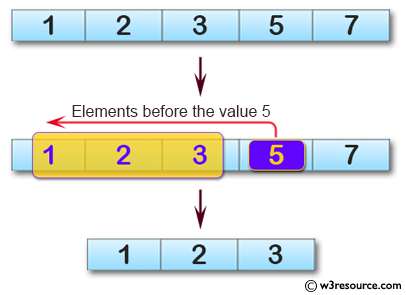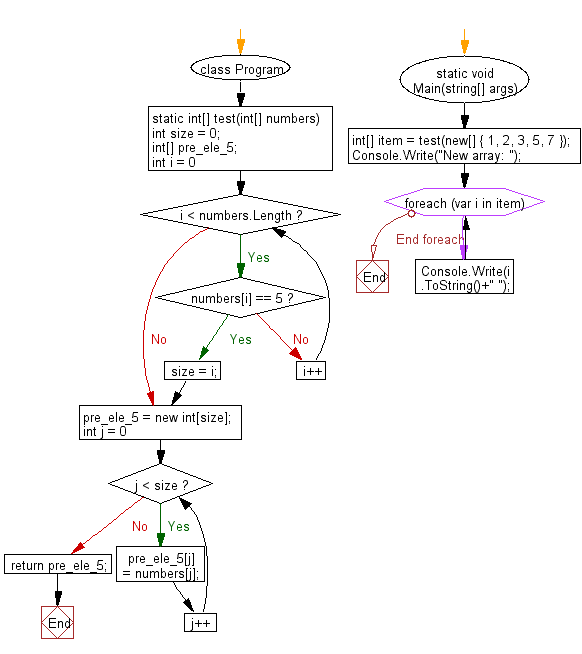﻿ C# - Array with the values before the value 5 from an array# C# Sharp Basic Algorithm Exercises: Create a new array taking the elements before the element value 5 from a given array of integers

## C# Sharp Basic Algorithm: Exercise-128 with Solution

Write a C# Sharp program to create a new array taking the elements before the element value 5 from a given array of integers.

Pictorial Presentation:Sample Solution:

C# Sharp Code:

``````using System;
namespace exercises
{
class Program
{
static void Main(string[] args)
{
int[] item = test(new[] { 1, 2, 3, 5, 7 });
Console.Write("New array: ");
foreach(var i in item)
{
Console.Write(i.ToString()+" ");
}
}
static int[] test(int[] numbers)
{
int size = 0;
int[] pre_ele_5;
for (int i = 0; i < numbers.Length; i++)
{
if (numbers[i] == 5)
{
size = i;
break;
}
}
pre_ele_5 = new int[size];

for (int j = 0; j < size; j++)
{
pre_ele_5[j] = numbers[j];
}
return pre_ele_5;
}
}
}
```
```

Sample Output:

`New array: 1 2 3`

Flowchart:C# Sharp Code Editor:

Improve this sample solution and post your code through Disqus

What is the difficulty level of this exercise?

Test your Programming skills with w3resource's quiz.

﻿